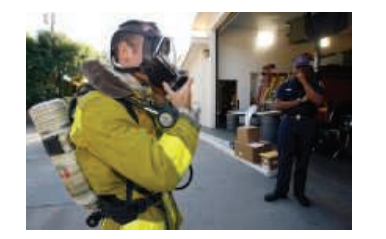×
Get Full Access to Chemistry: The Central Science - 14 Edition - Chapter 3 - Problem 3.62
Get Full Access to Chemistry: The Central Science - 14 Edition - Chapter 3 - Problem 3.62

×

# ?The reaction between potassium superoxide, $$\mathrm{KO}_{2}$$, and $$\mathrm{CO}_{2}$$, $$4 \mathrm{KO}_{2}+2 \mathrm{CO}_{2} \longrightarroISBN: 9780134414232 1274 ## Solution for problem 3.62 Chapter 3 Chemistry: The Central Science | 14th Edition • Textbook Solutions • 2901 Step-by-step solutions solved by professors and subject experts • Get 24/7 help from StudySoup virtual teaching assistantsChemistry: The Central Science | 14th Edition 4 5 1 263 Reviews 24 3 Problem 3.62 The reaction between potassium superoxide, \(\mathrm{KO}_{2}$$, and $$\mathrm{CO}_{2}$$,

$$4 \mathrm{KO}_{2}+2 \mathrm{CO}_{2} \longrightarrow 2 \mathrm{~K}_{2} \mathrm{CO}_{3}+3 \mathrm{O}_{2}$$

is used as a source of $$\mathrm{O}_{2}$$ and absorber of $$\mathrm{CO}_{2}$$ in self contained breathing equipment used by rescue workers.

(a) How many moles of $$\mathrm{O}_{2}$$ are produced when 0.400 mol of $$\mathrm{KO}_{2}$$ reacts in this fashion?

(b) How many grams of $$\mathrm{KO}_{2}$$ are needed to form 7.50 g of $$\mathrm{O}_{2}$$ ?(c) How many grams of $$\mathrm{CO}_{2}$$ are used when 7.50 g of $$\mathrm{O}_{2}$$ are produced?

Text Transcription:

KO2

CO2

4 KO2 + 2 CO2 \longrightarrow 2K2CO3 + 3 O2

O2

Step-by-Step Solution:

Step 1 of 5) How many moles of O2 are produced when 0.400 mol of KO2 reacts in this fashionHow many grams of KO2 are needed to form 7.50 g of O2 Solution Stoichiometry and Chemical Analysis In Chapter 3 we learned that given the chemical equation for a reaction and the amount of one reactant consumed in the reaction, you can calculate the quantities of other reactants and products. In this section we extend this concept to reactions involving solutions. Recall that the coefficients in a balanced equation give the relative number of moles of reactants and products. (Section 3.6) To use this information, we must convert the masses of substances involved in a reaction into moles. When dealing with pure substances, as we did in Chapter 3, we use molar mass to convert between grams and moles of the substances.

Step 2 of 2

## Discover and learn what students are asking

Calculus: Early Transcendental Functions : Evaluating Limits Analytically
?Finding a Limit In Exercises 5-36, find the limit. $$\lim _{x \rightarrow-3} x^{4}$$

Calculus: Early Transcendental Functions : Inverse Trigonometric Functions: Integration
?In Exercises 1-20, find the indefinite integral. $$\int \frac{1}{\sqrt{1-(x+1)^{2}}} d x$$

Calculus: Early Transcendental Functions : Differential Equations: Growth and Decay
?In Exercises 1-10, solve the differential equation. $$y^{\prime}=\frac{5 x}{y}$$

Calculus: Early Transcendental Functions : Differentiation and Integration of Vector-Valued Functions
?Differentiation of Vector-Valued Functions In Exercises 1-6, find r’(t), $$\mathrm{r}\left(t_{0}\right)$$, and $$\mathrm{r’}\left(t_{0}\right)$$ for t

Statistics: Informed Decisions Using Data : Comparing Three or More Means (One-Way Analysis of Variance)
?In Problems 7 and 8, fill in the ANOVA table.

Unlock Textbook Solution

?The reaction between potassium superoxide, $$\mathrm{KO}_{2}$$, and $$\mathrm{CO}_{2}$$, \(4 \mathrm{KO}_{2}+2 \mathrm{CO}_{2} \longrightarro